Reactivity in Chemistry

Carbonyl Addition

CO28.  Solutions to Selected Problems, Part B

Problem CO13.1.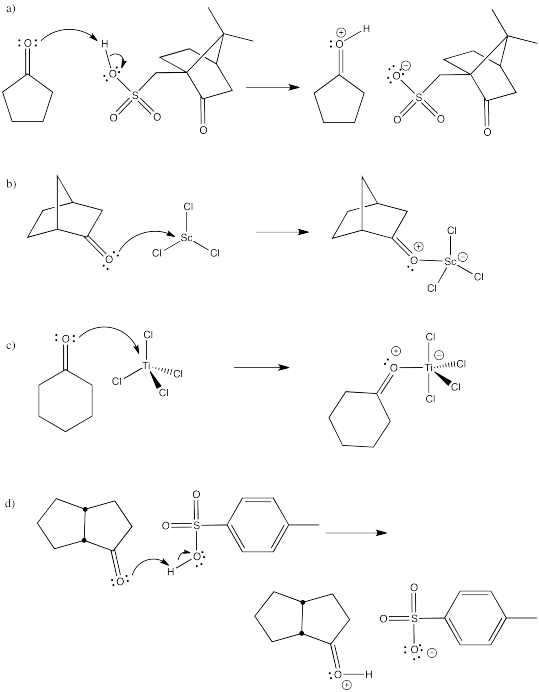Problem CO13.2.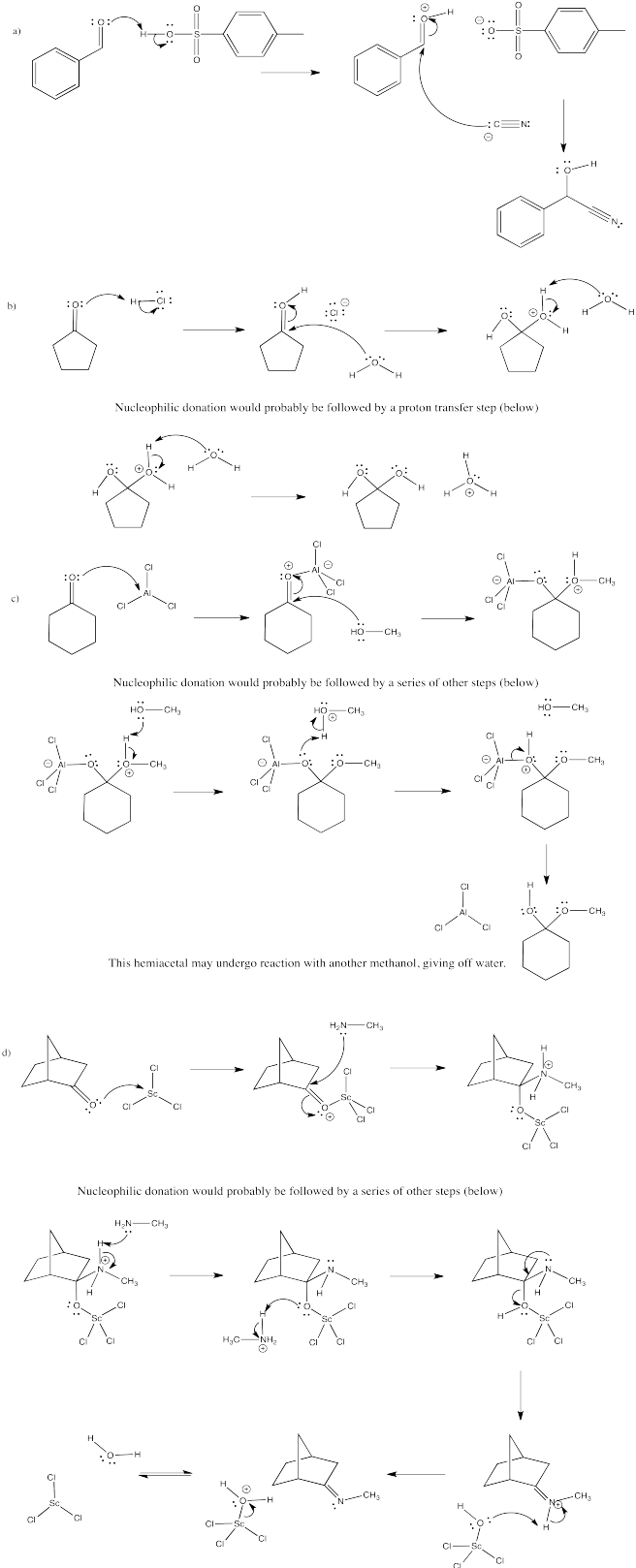Problem CO13.3.

Part a.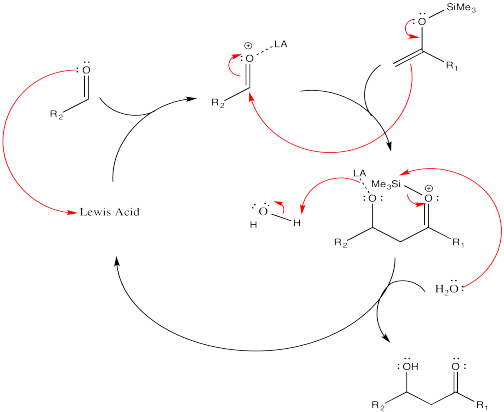Part b.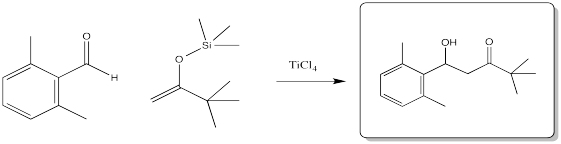Problem CO13.4.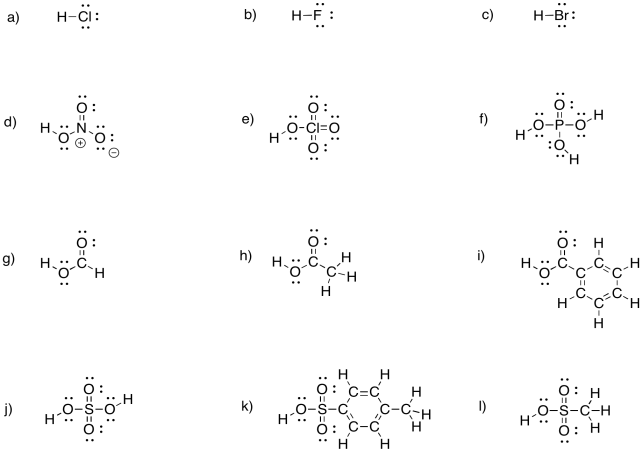Problem CO14.1.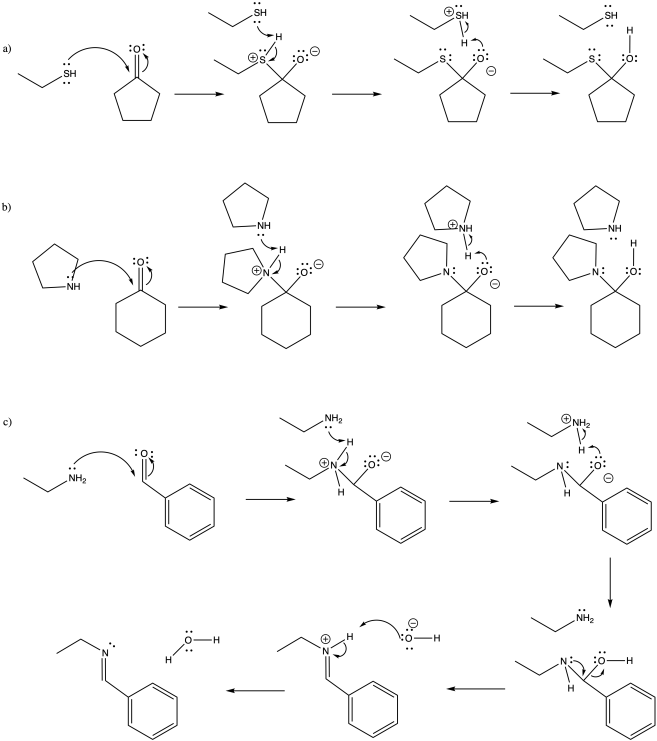Problem CO14.2.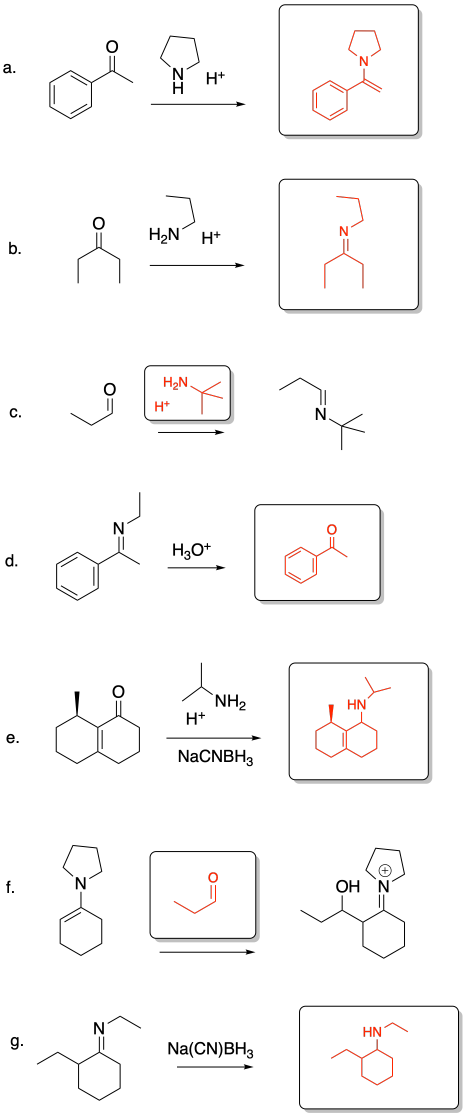Problem CO14.3.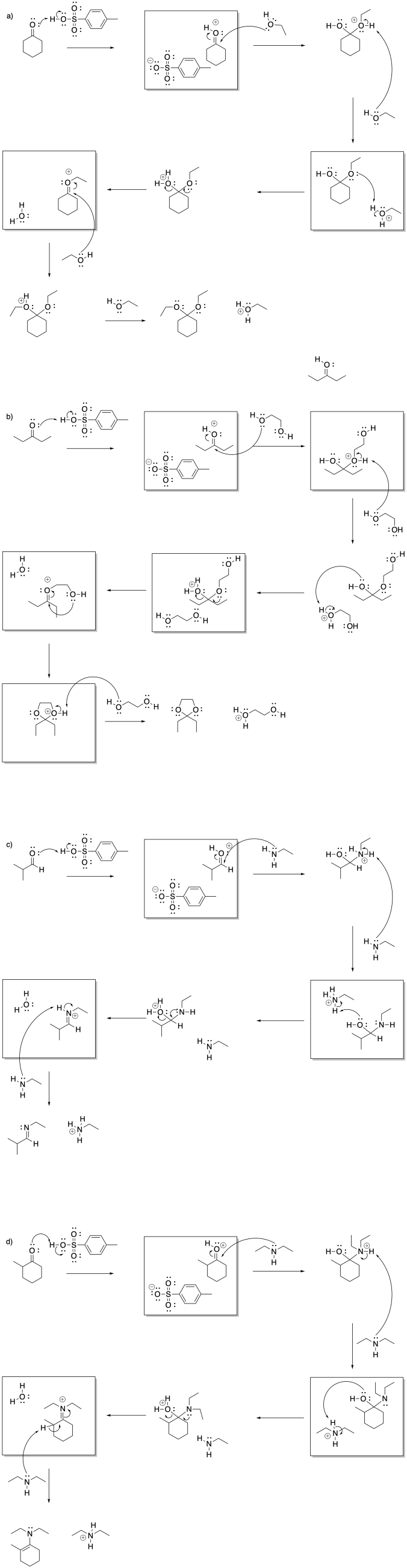Problem CO14b.1.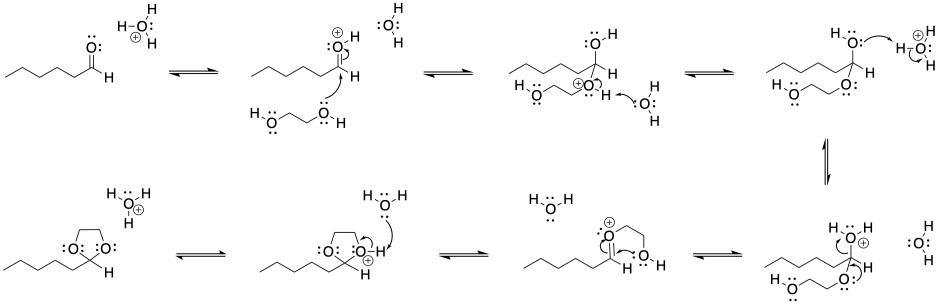Problem CO14b.2.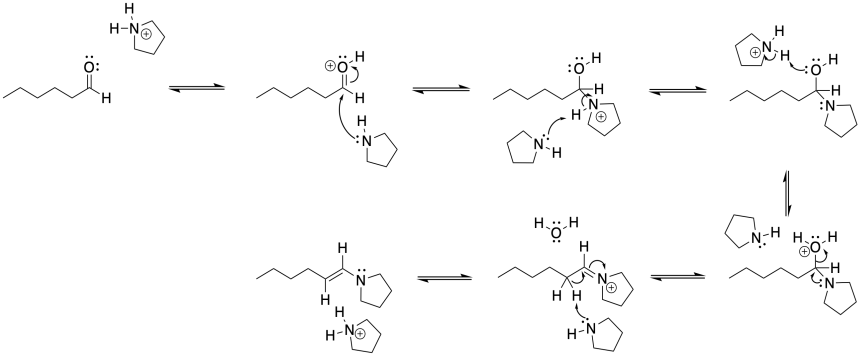Problem CO14b.3.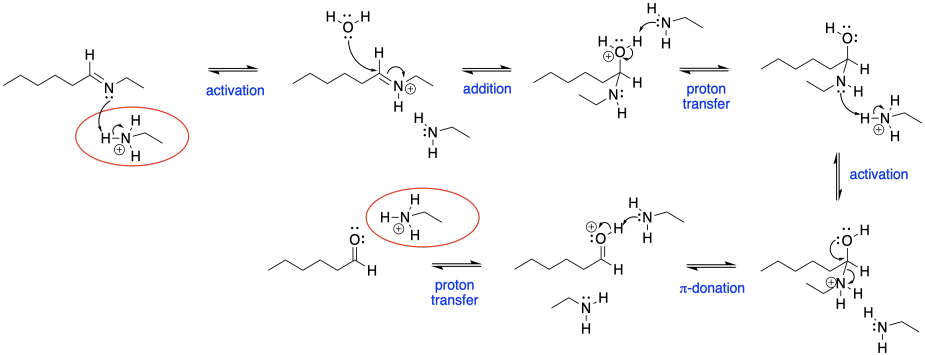Problem CO14b.4.

This mechanism requires formation of both an iminium ion (blue) and an enol (red).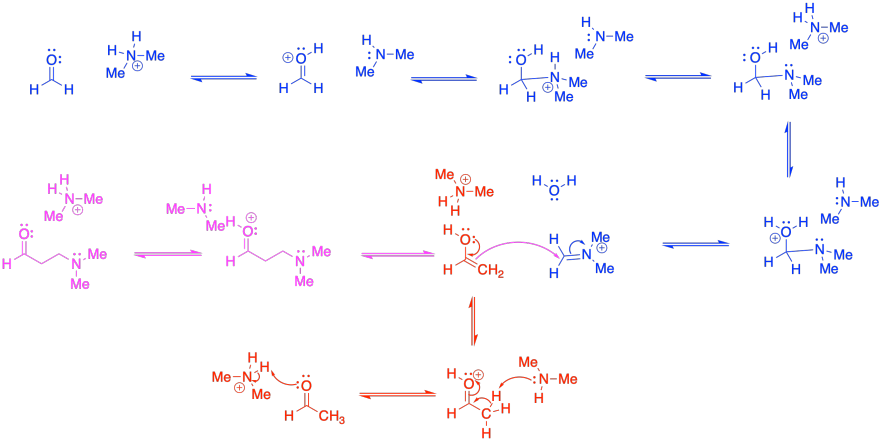Problem CO15.1.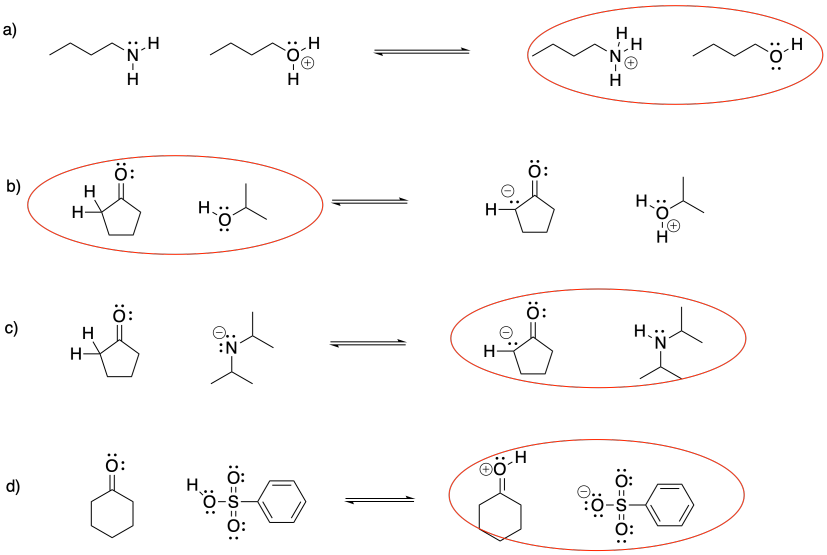Problem CO15.2.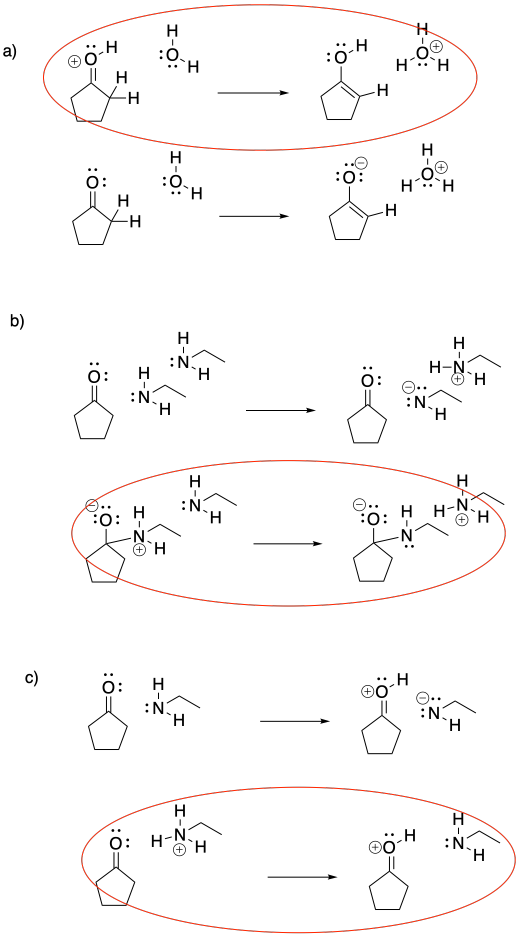Problem CO16.1.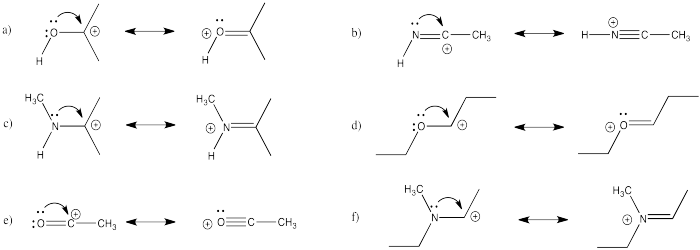Problem CO16.2.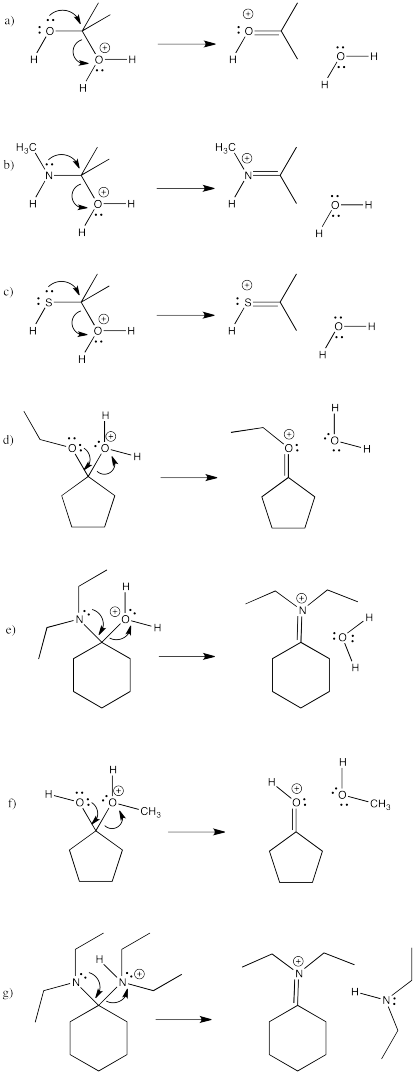Problem CO16.3.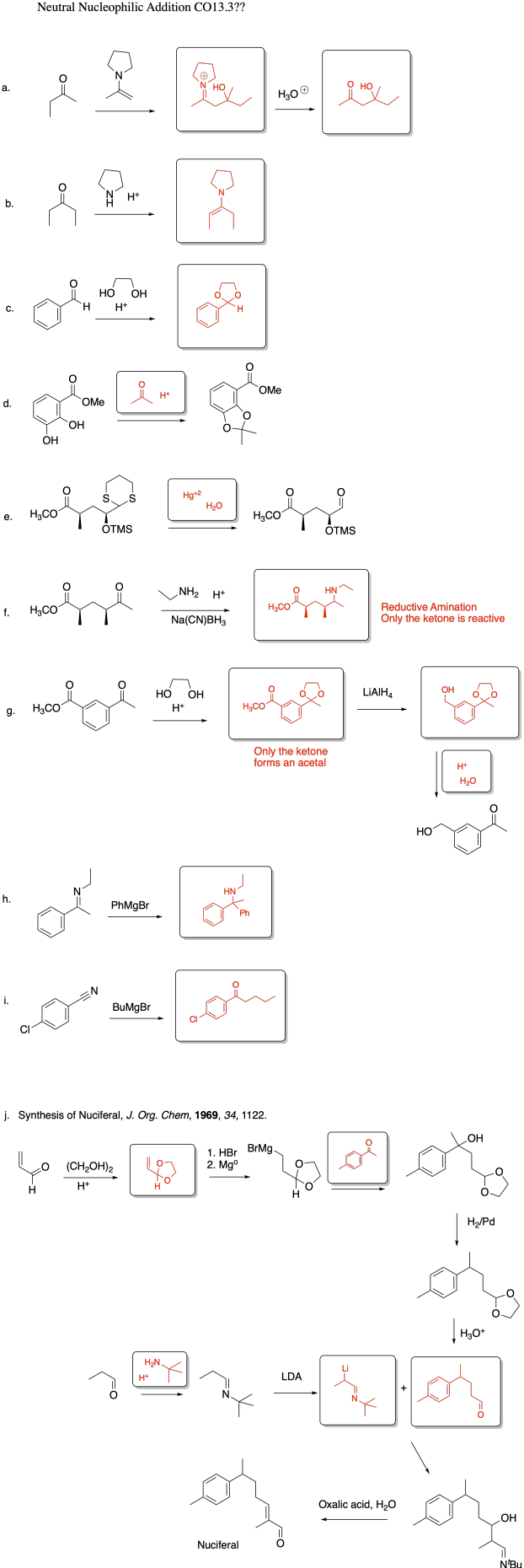Problem CO18.1.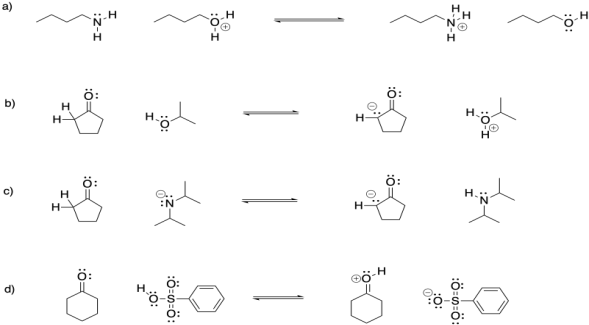Problem CO18.2.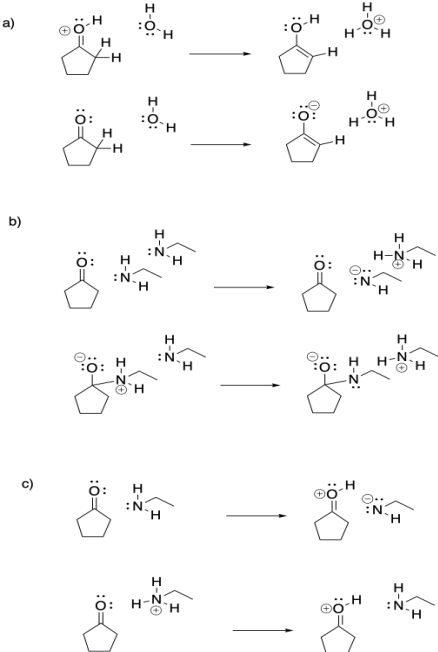Problem CO18.4.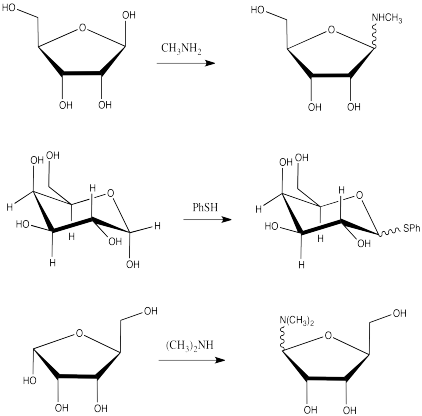Problem CO18.5.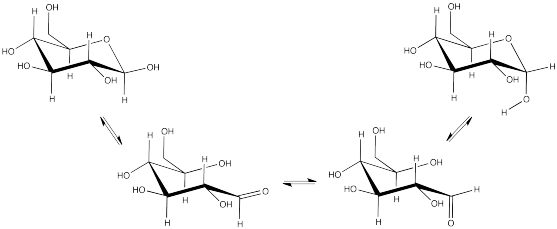Problem CO18.7.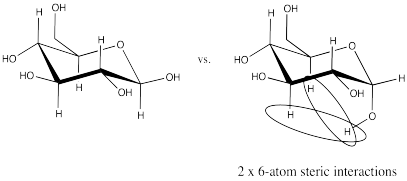Problem CO18.8.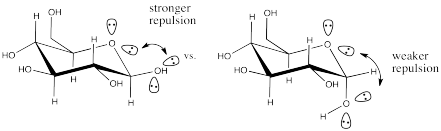Problem CO19.1.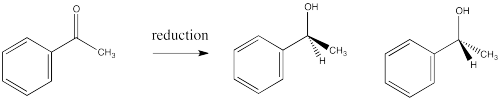Problem CO20.6.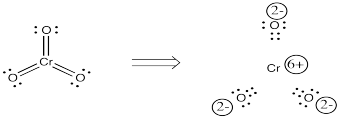Problem CO20.7.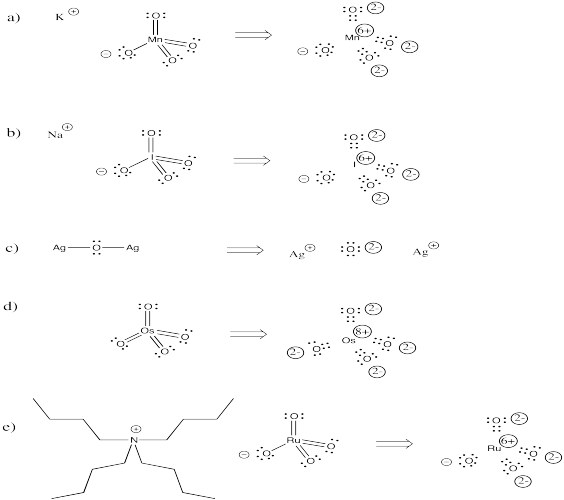Problem CO21.1.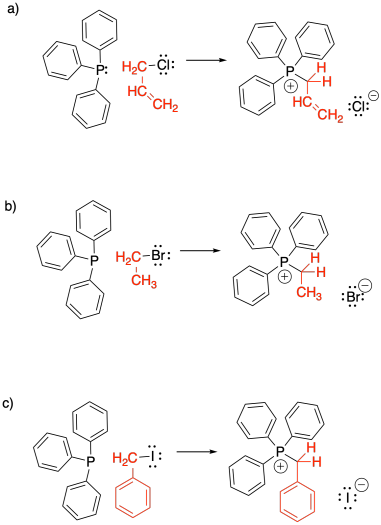Problem CO21.2.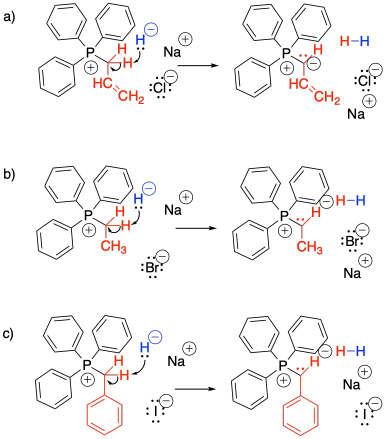Problem CO21.3.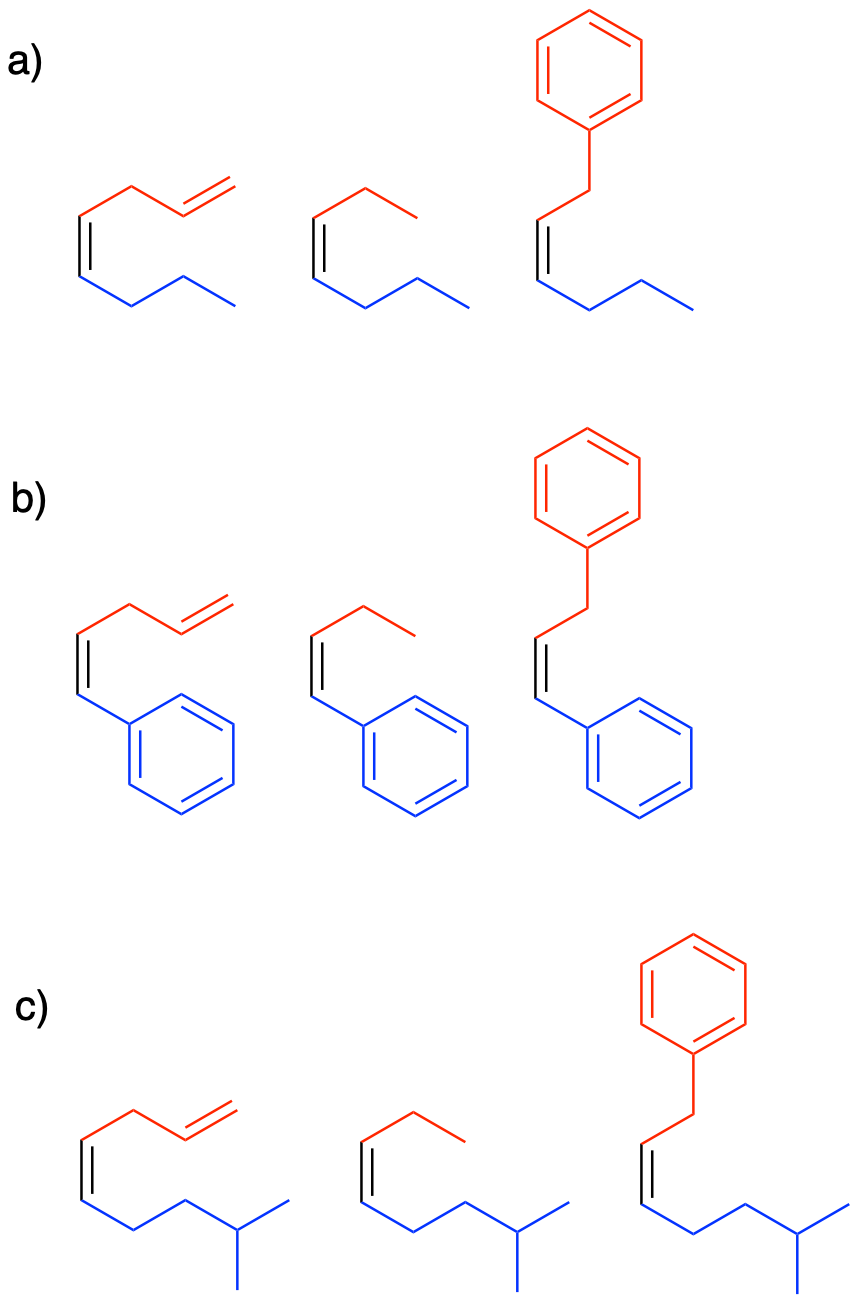Problem CO21.4.

One of the keys in this problem is recognizing that in some steps, two different reactions are involved.  For example, in the first box, there is an addition of a diol to a carbonyl followed by an ylide addition.

Problem CO21.5.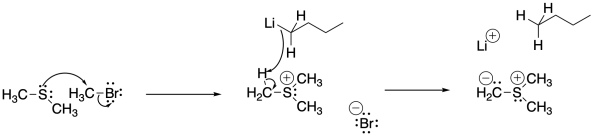Problem CO21.6.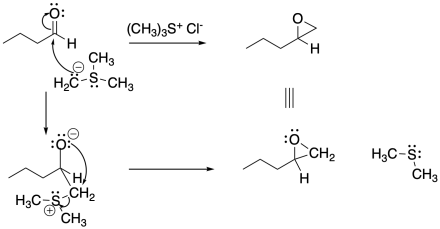Problem CO21.7.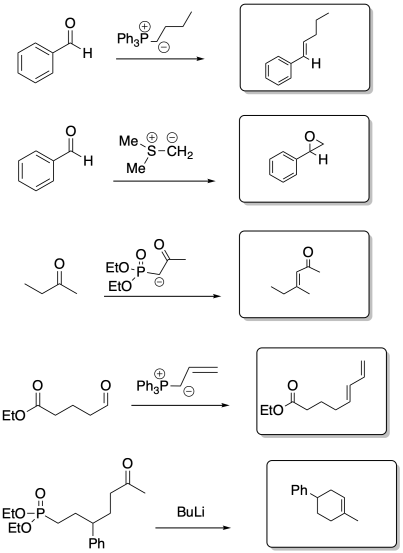Problem CO21.8.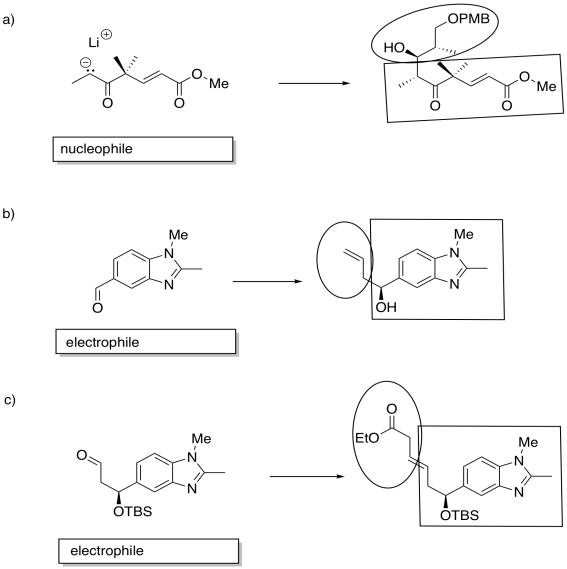Problem CO21.9.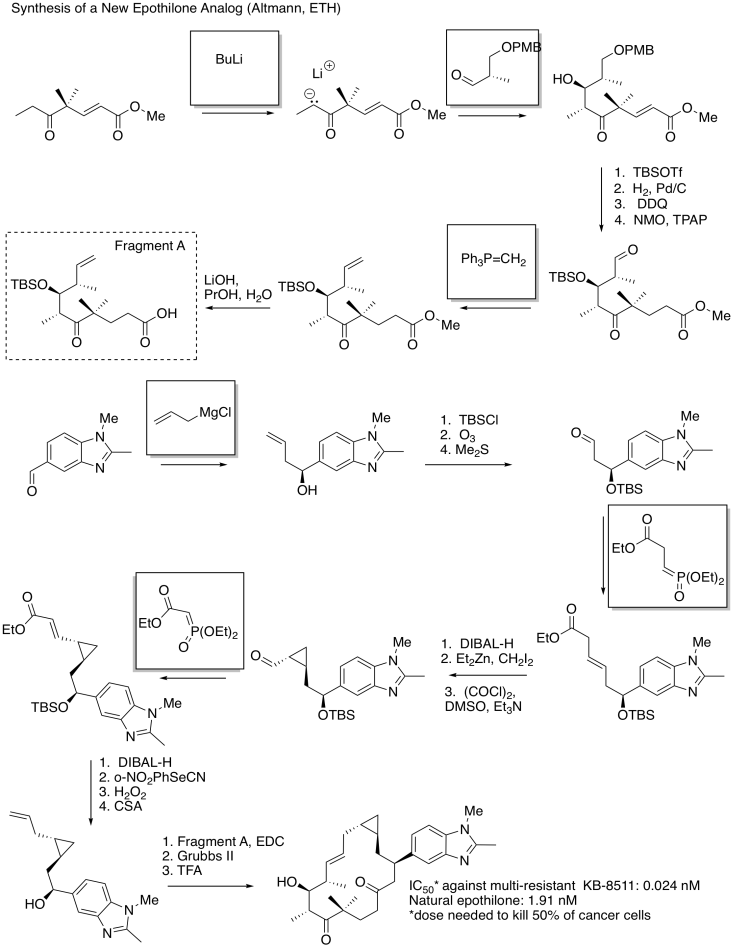Problem CO21.10.

a)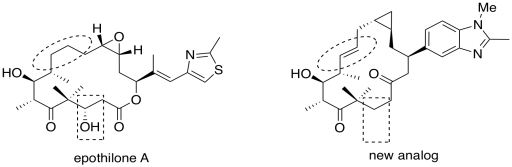b) The circled part changed from a single bond to a doube bond.  The boxed part changed from an alcohol to unadorned hydrocarbon chain.

c) Hydrogen bonding is the most obvious.

d) However, the analog works better without this group; this particular alcohol group is probably not an important part of the pharmacophore.  It is probably not needed in order to bind to the target.

e) In epothilone A, as drawn, the dihedral angle appears to be 0 degrees.

f) In the new analog, the dihedral angle is 180 degrees.

g) Based on the superior activity of the analog, the active conformation of the ring is probably more like the one on the right than the one on the left.  The circled bond probably adopts a dihedral angle closer to 180 degrees, with the rest of the ring twisting into a shape more like the one shown on the right, in order to bind to the target.

Problem CO22.1.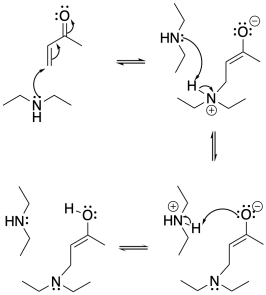Problem CO22.2.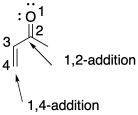Problem CO22.3.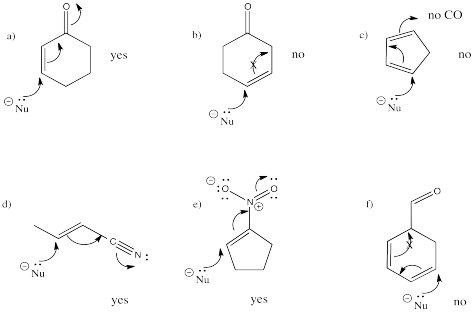Problem CO22.4.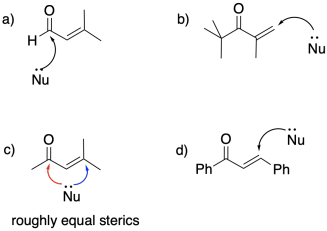Problem CO22.6.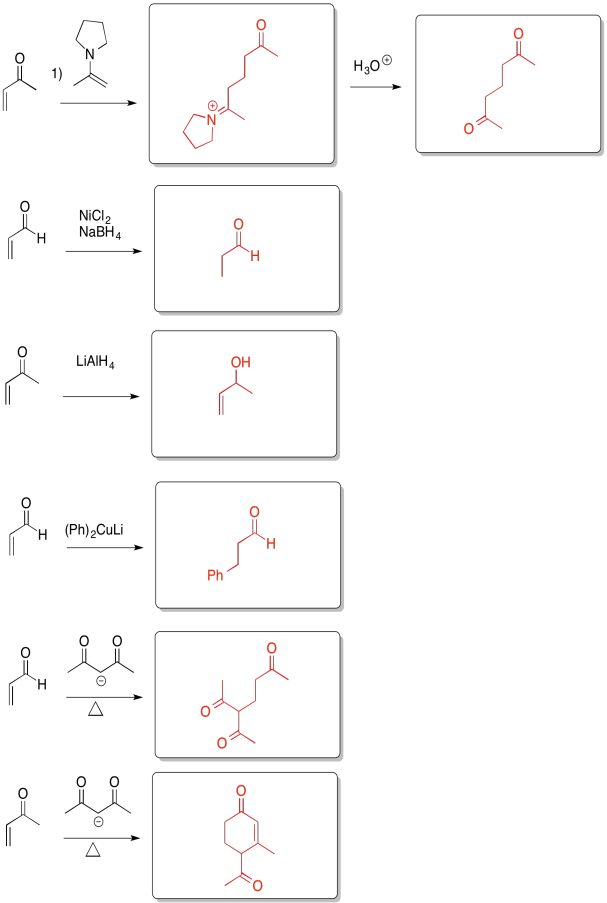Problem CO22.7.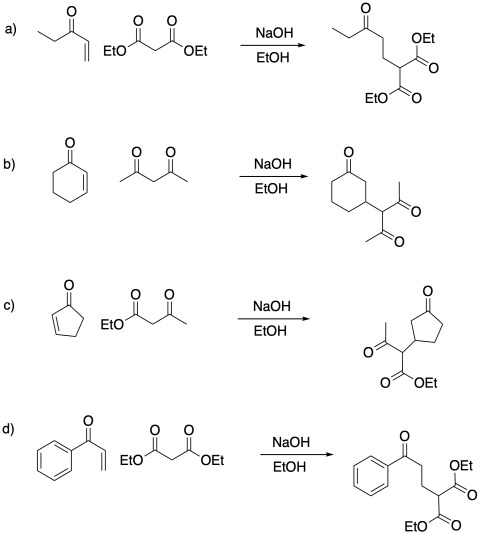Problem CO22.8.

a)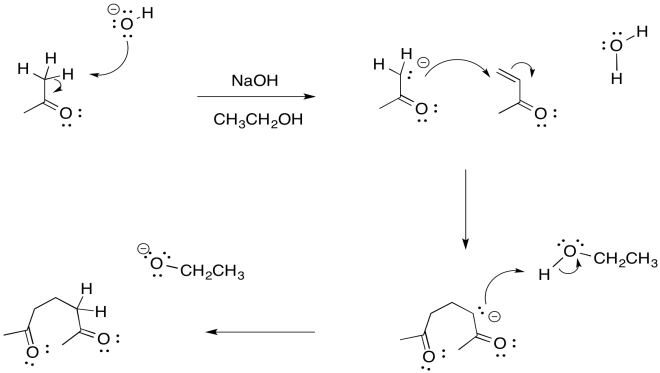b)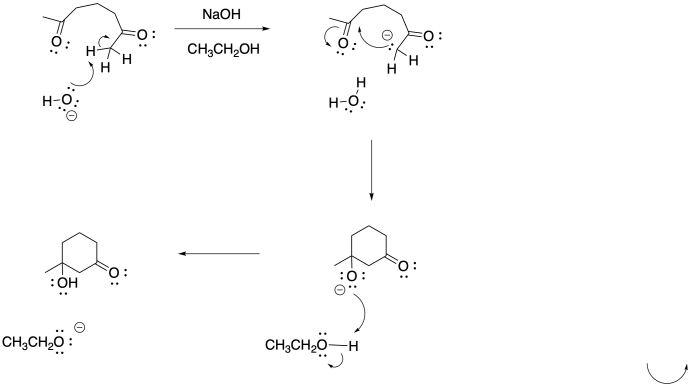c)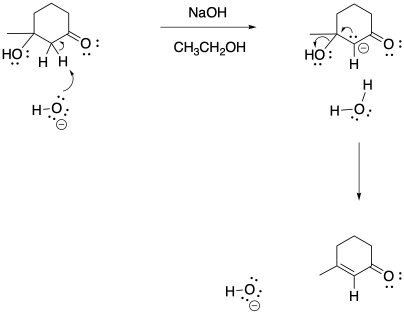Problem CO22.9.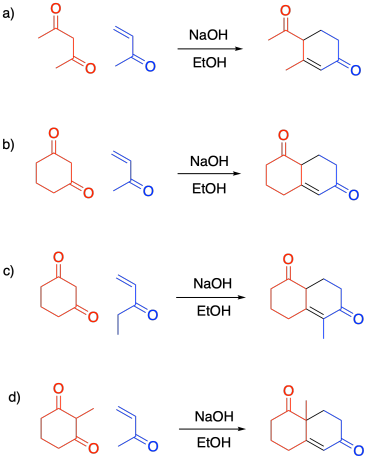Problem CO22.10.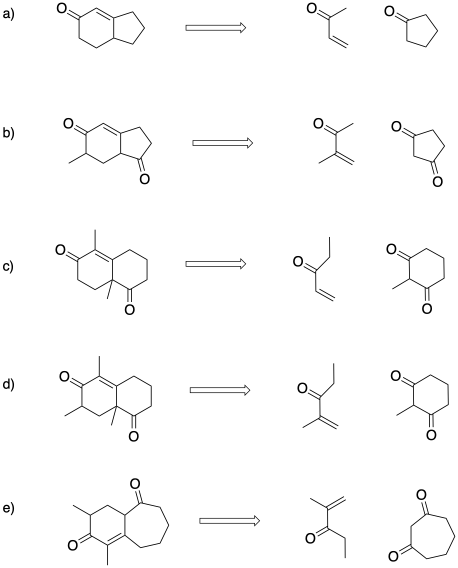Problem CO23.1.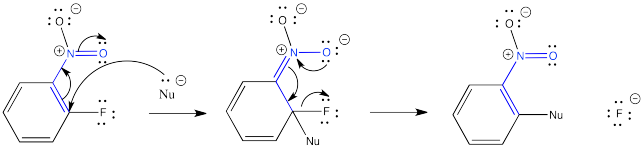Problem CO24.1.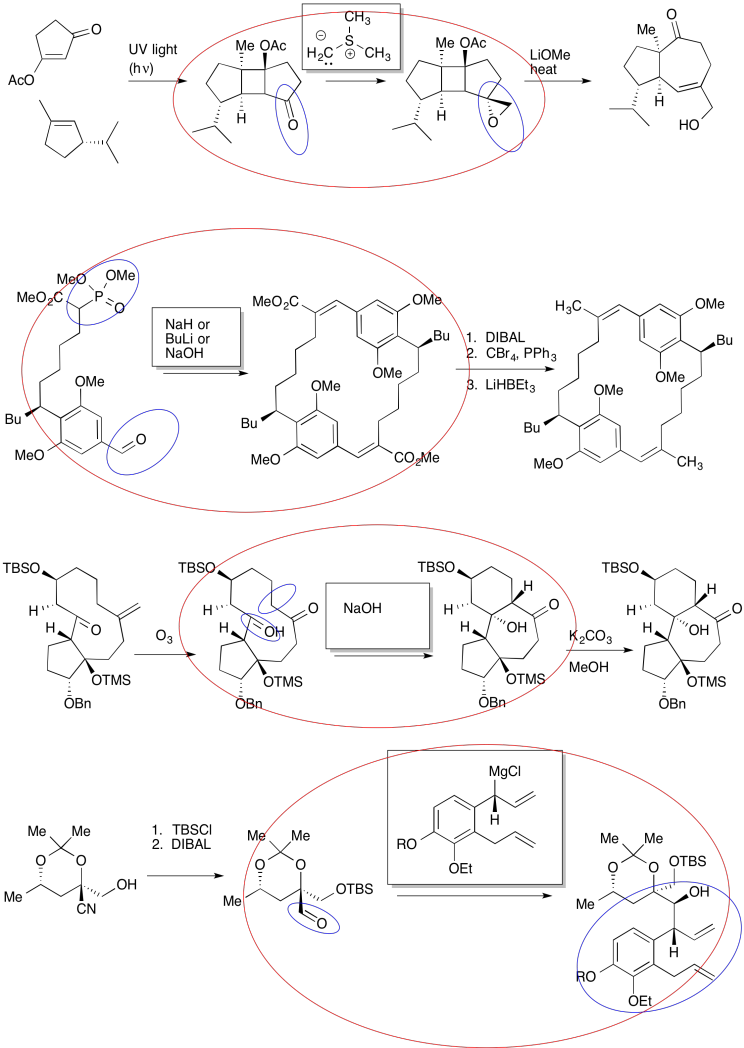Problem CO24.2.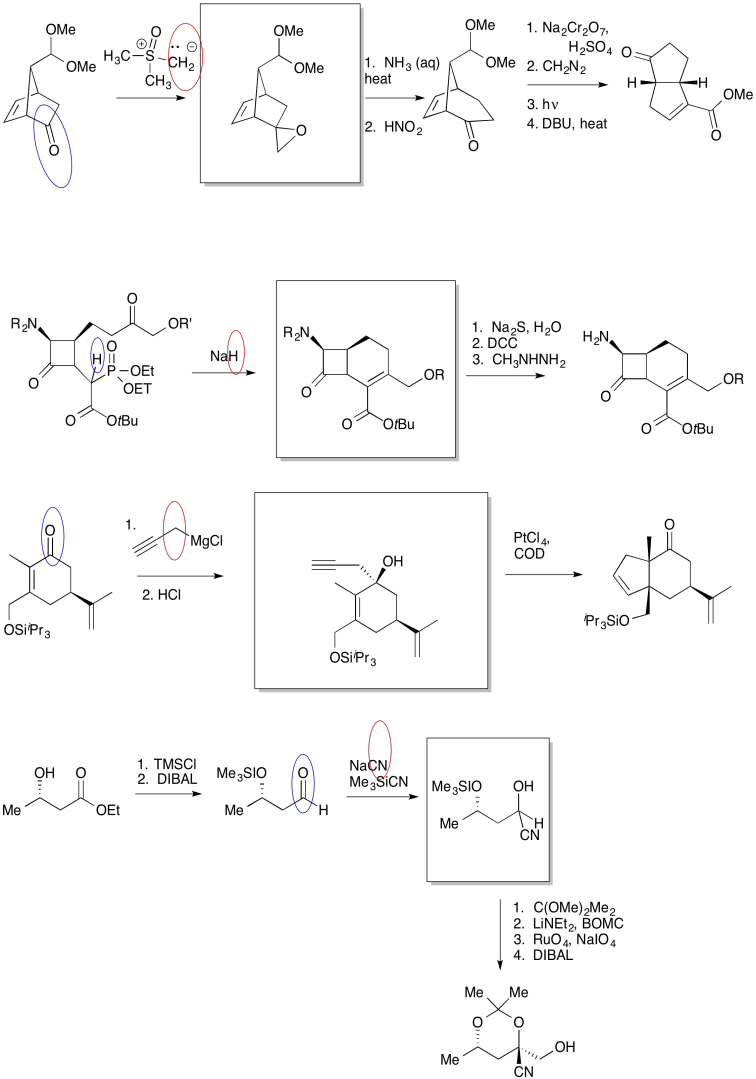Problem CO24.3.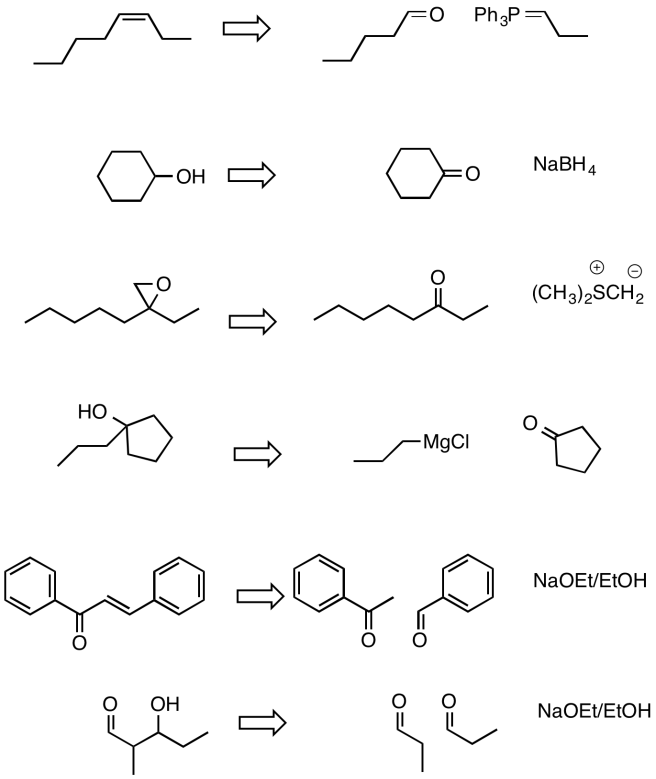Problem CO24.4.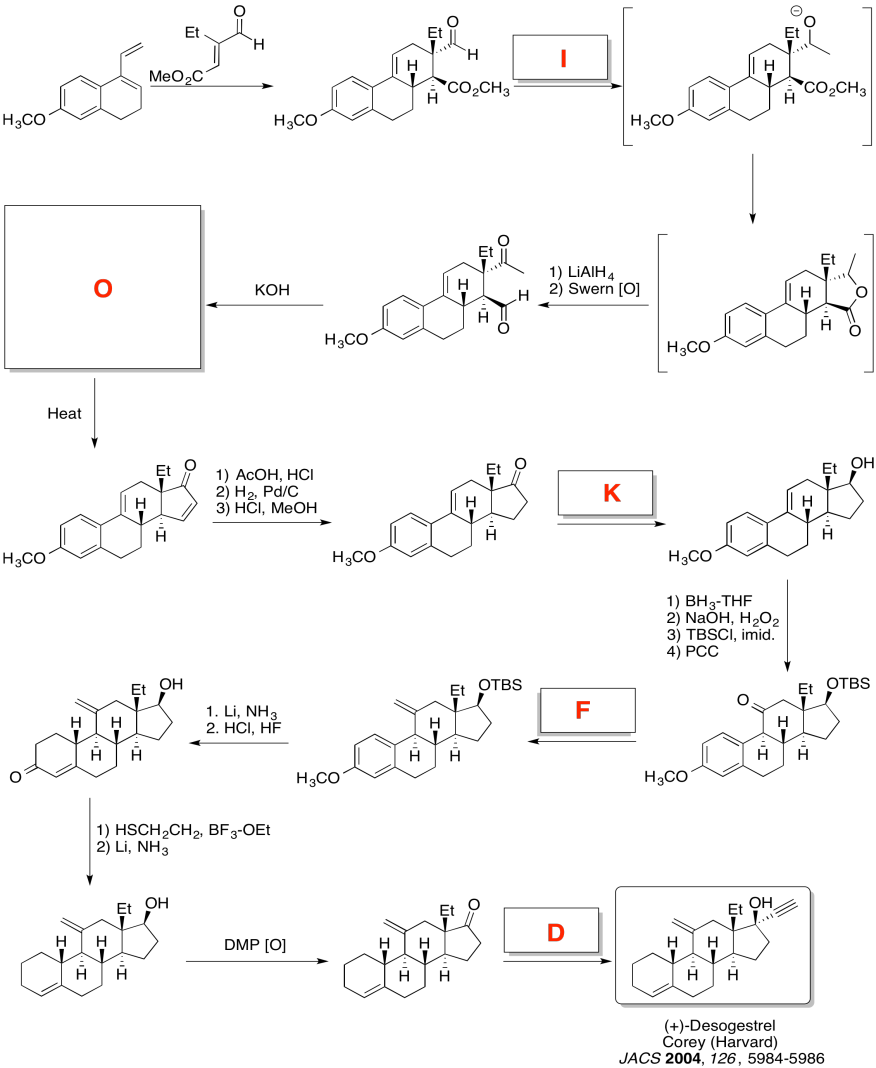Problem CO24.5.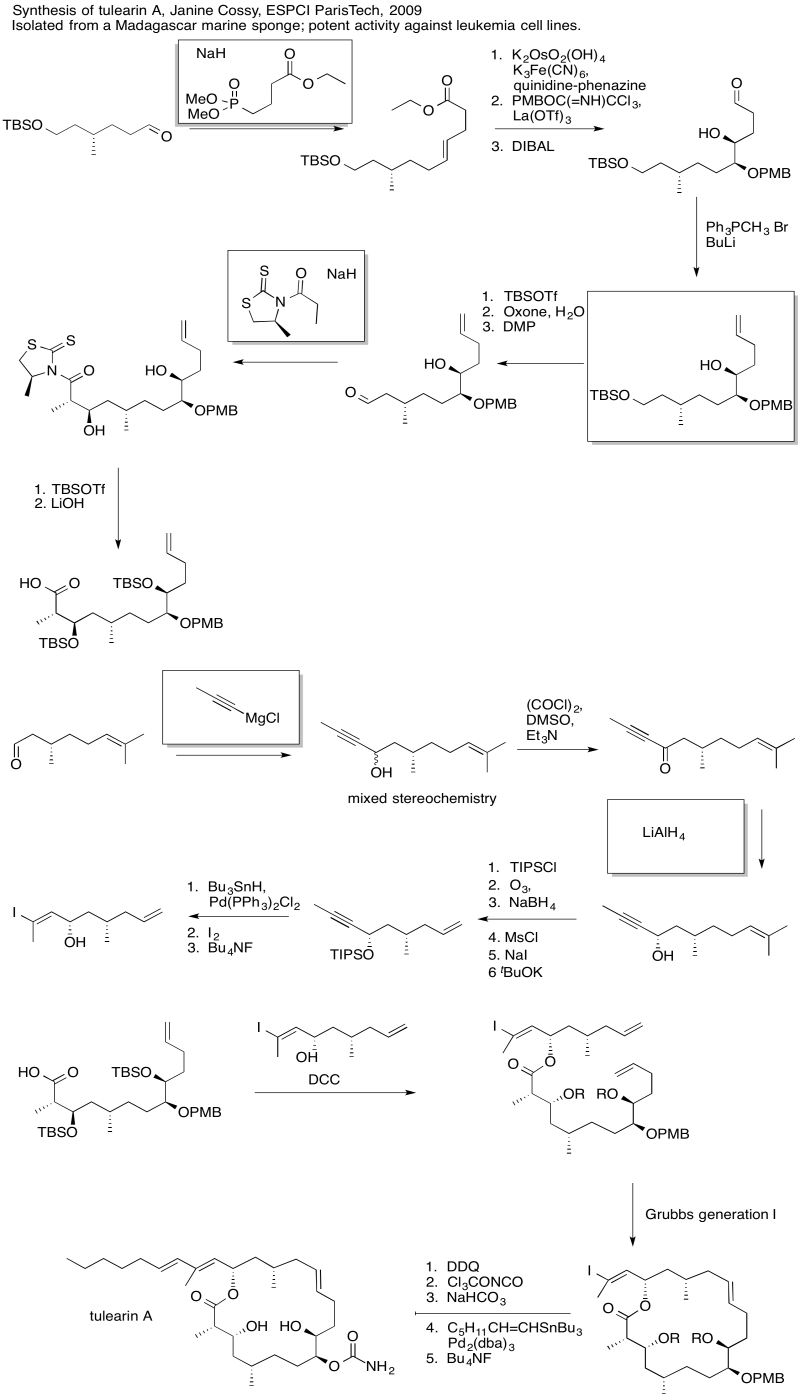Problem CO24.6.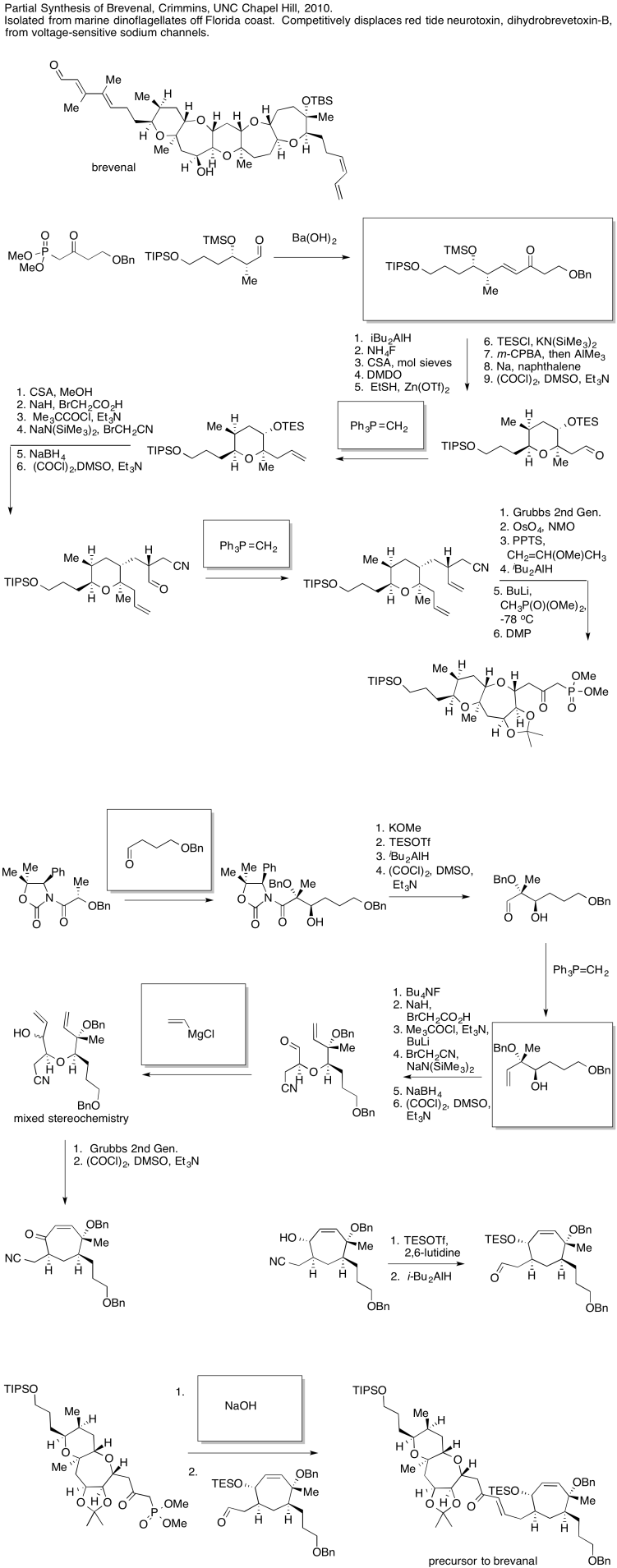Problem CO26.1.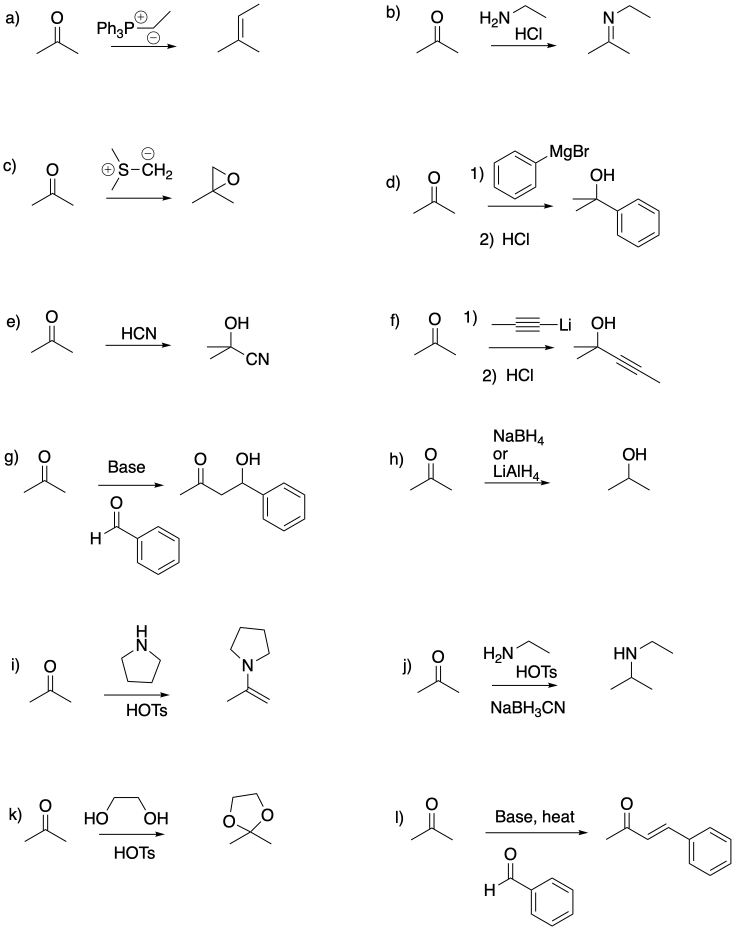Problem CO26.2.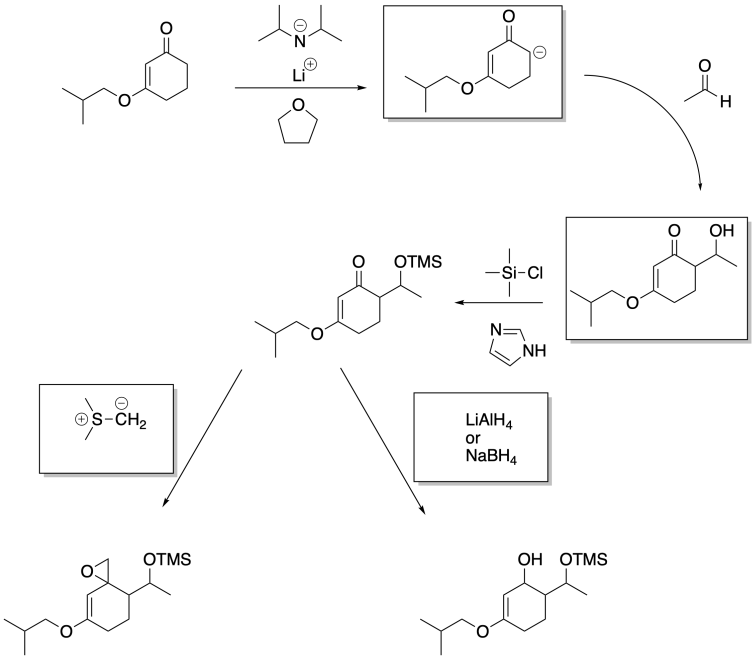Problem CO26.3.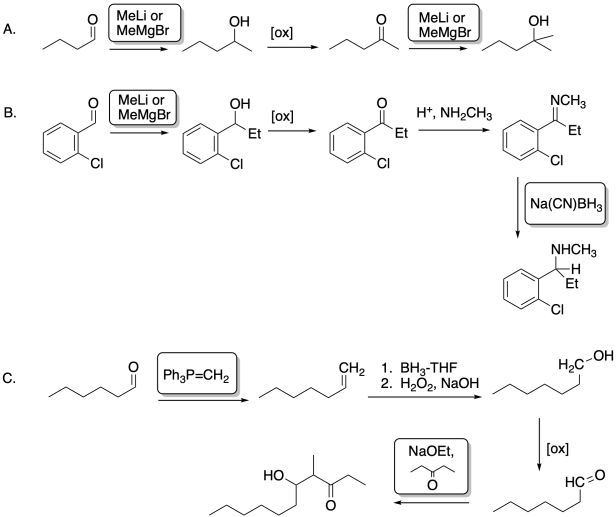Problem CO26.4.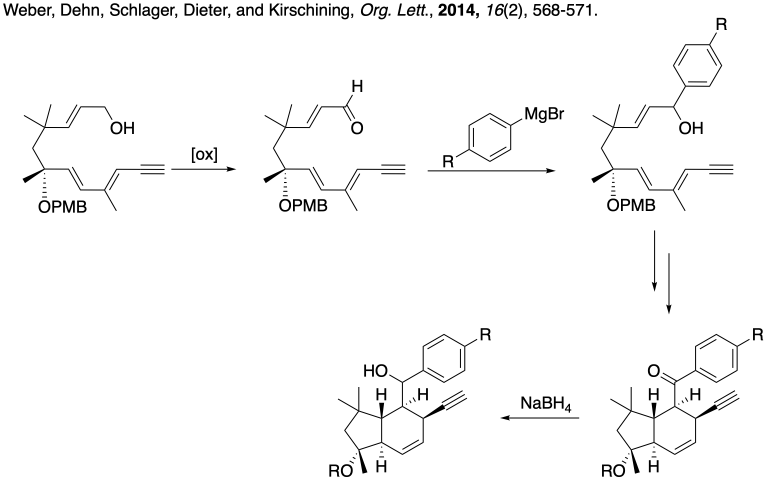Problem CO26.5.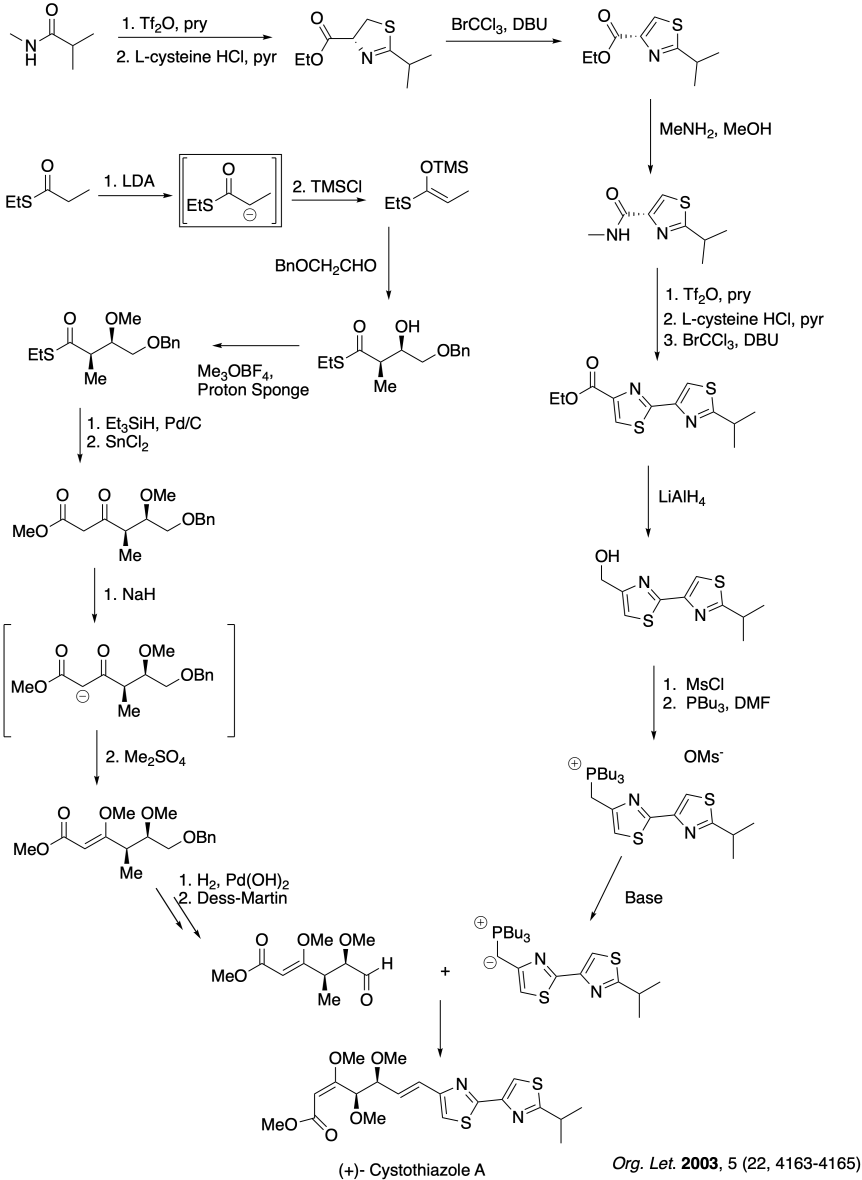Problem CO26.6.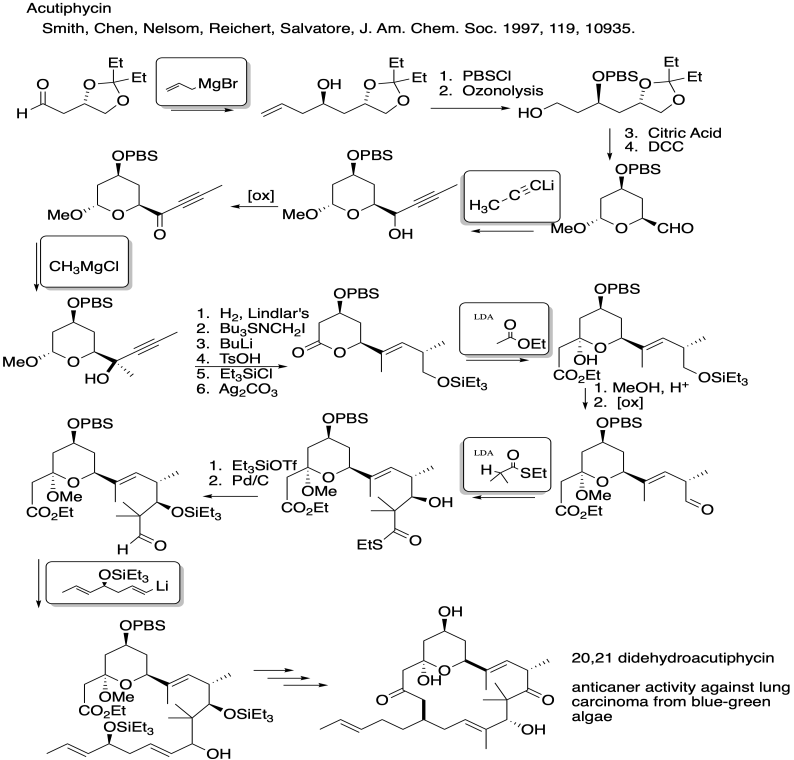Problem CO26.7.Problem CO26.8.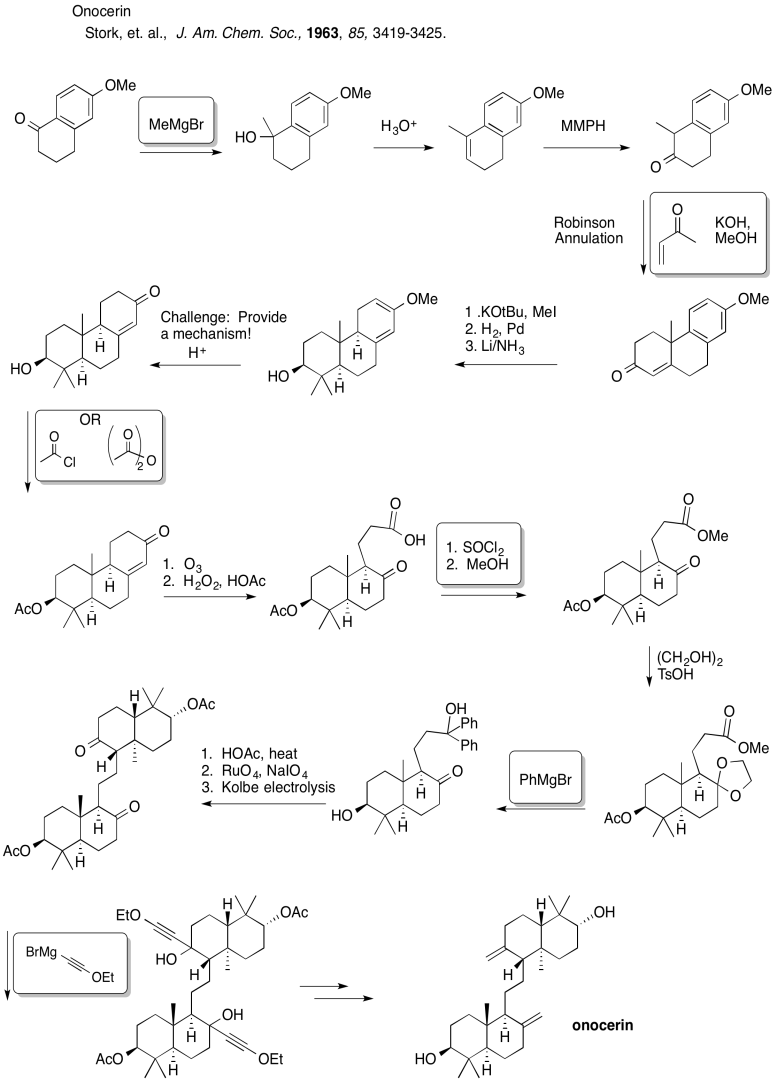Problem CO26.9.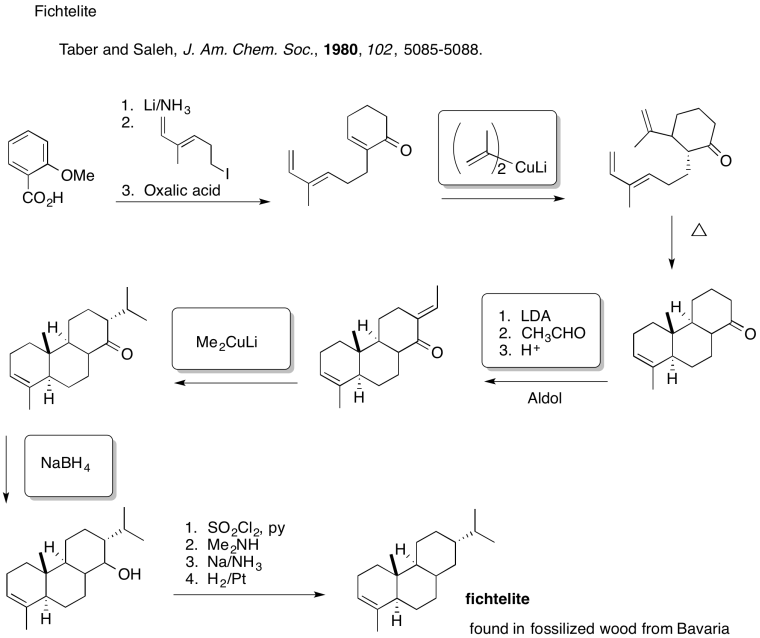This site is written and maintained by Chris P. Schaller, Ph.D., College of Saint Benedict / Saint John's University (with contributions from other authors as noted).  It is freely available for educational use.Structure & Reactivity in Organic, Biological and Inorganic Chemistry by Chris Schaller is licensed under a Creative Commons Attribution-NonCommercial 3.0 Unported License

Send corrections to cschaller@csbsju.edu

This material is based upon work supported by the National Science Foundation under Grant No. 1043566.

Any opinions, findings, and conclusions or recommendations expressed in this material are those of the author(s) and do not necessarily reflect the views of the National Science Foundation.

Navigation:

Back to Web Materials on Structure & Reactivity in Chemistry